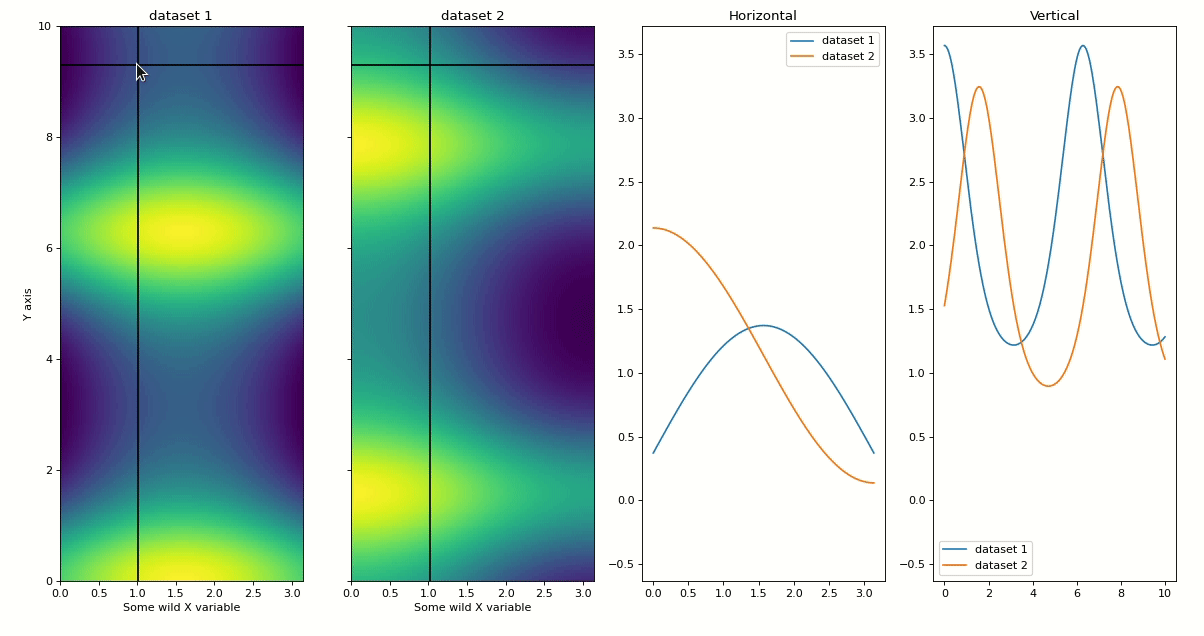# Heatmap Slicer#

%matplotlib widget
import matplotlib.pyplot as plt
import numpy as np

from mpl_interactions import heatmap_slicer


## Comparing heatmaps#

Sometimes I find myself wanting to compare horizontal or vertical slices across two different heatmaps with the same shape. The function heatmap_slicer() makes this easy and should work for any number of heatmaps from 1 to many (likely not all the way $$\infty$$ though).

The most important options to play with are:

• slices: "both", "vertical", or "horizontal"

• interaction_type: "move" or "click"

x = np.linspace(0, np.pi, 100)
y = np.linspace(0, 10, 200)
X, Y = np.meshgrid(x, y)
data1 = np.sin(X) + np.exp(np.cos(Y))
data2 = np.cos(X) + np.exp(np.sin(Y))
fig, axes = heatmap_slicer(
x,
y,
(data1, data2),
slices="both",
heatmap_names=("dataset 1", "dataset 2"),
labels=("Some wild X variable", "Y axis"),
interaction_type="move",
)